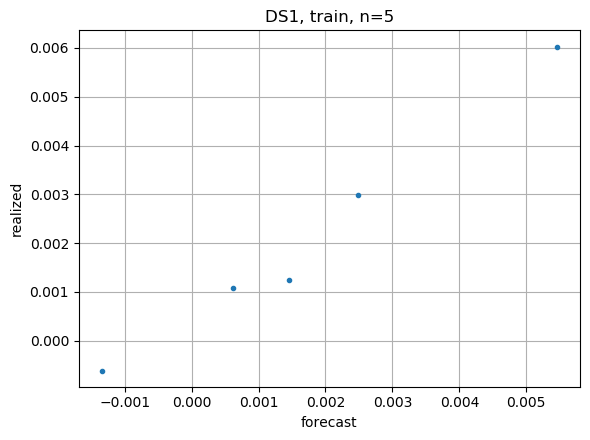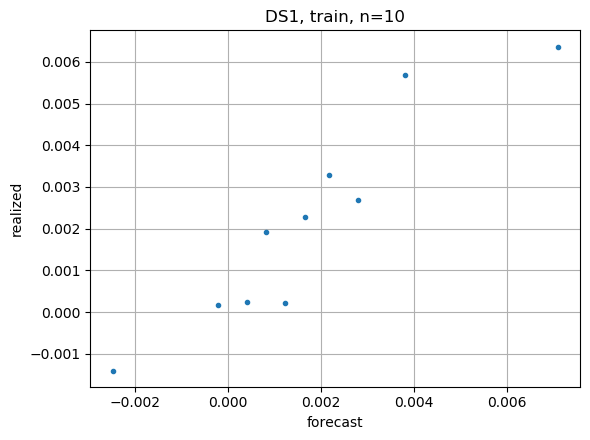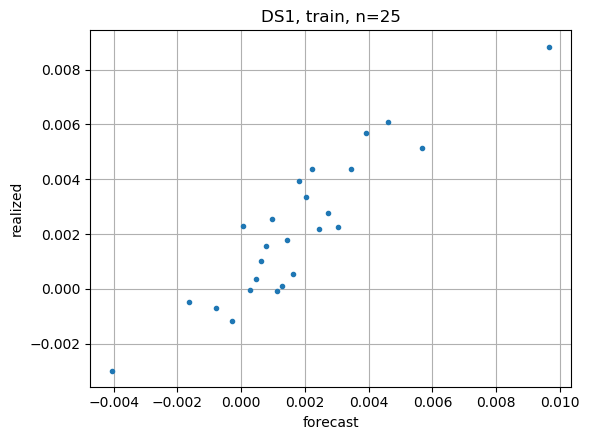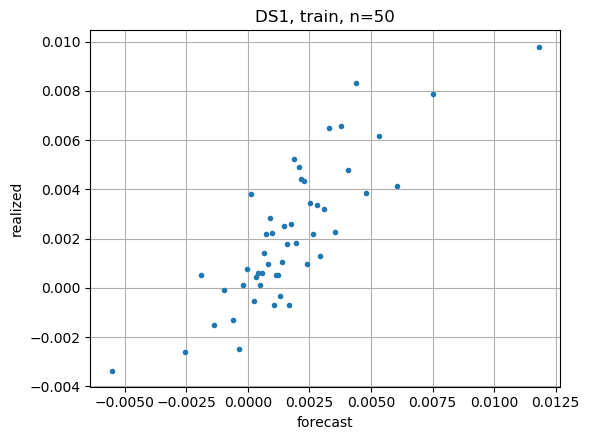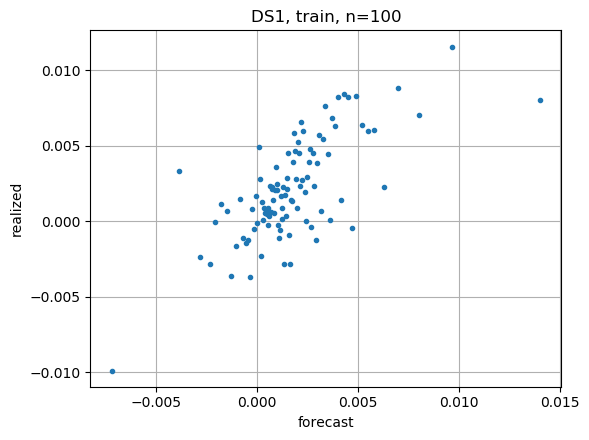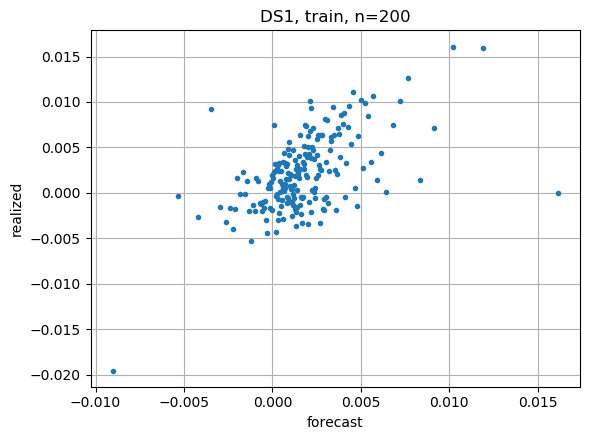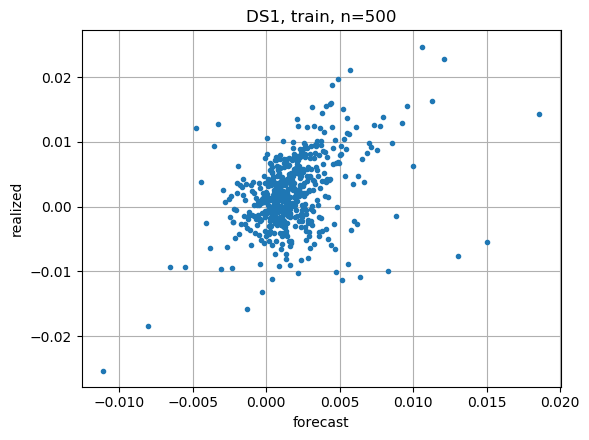# Binned FVR

## Forecast vs. realized

FVR plots are useful for visualizing how well a model fits the data as the strength of the model forecast changes. Plots made on training data should show a roughly linear relationship, with slope=1. Plots made on test data usually show a weaker relationship, as the effects of overfitting are exposed.

Judging model quality is as much art as science. Of course we use metrics such as $R^2$ in situations where we need to compare models automatically, such as during cross-validation. But the FVR plot shows so much more information. For example, for some datasets I don’t really care if my tiny forecasts near the origin are correct or not, since I won’t be trading those. I do care a lot about my forecasts far from the origin, as that is where I can overcome transaction costs and make money. I prefer when my large forecasts appear conservative. And I want my model to make lots of large forecasts!

## Binning

Financial datasets tend to be large and extremely noisy. Raw scatterplots are useless, as the dots fill the plot area, and the clutter masks the structure. So, I prefer making binned scatterplots, where each dot represents the weighted average location of points in the bin. Here are examples from my handcrafted model on dataset-1 with varying numbers of bins. You can see how using fewer dots brings out the structure in the scatterplots: